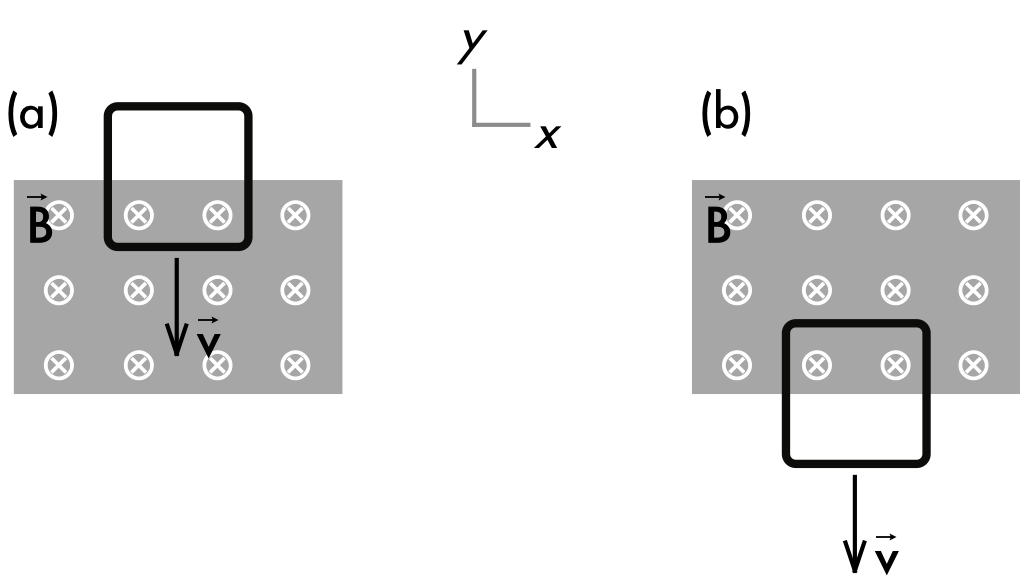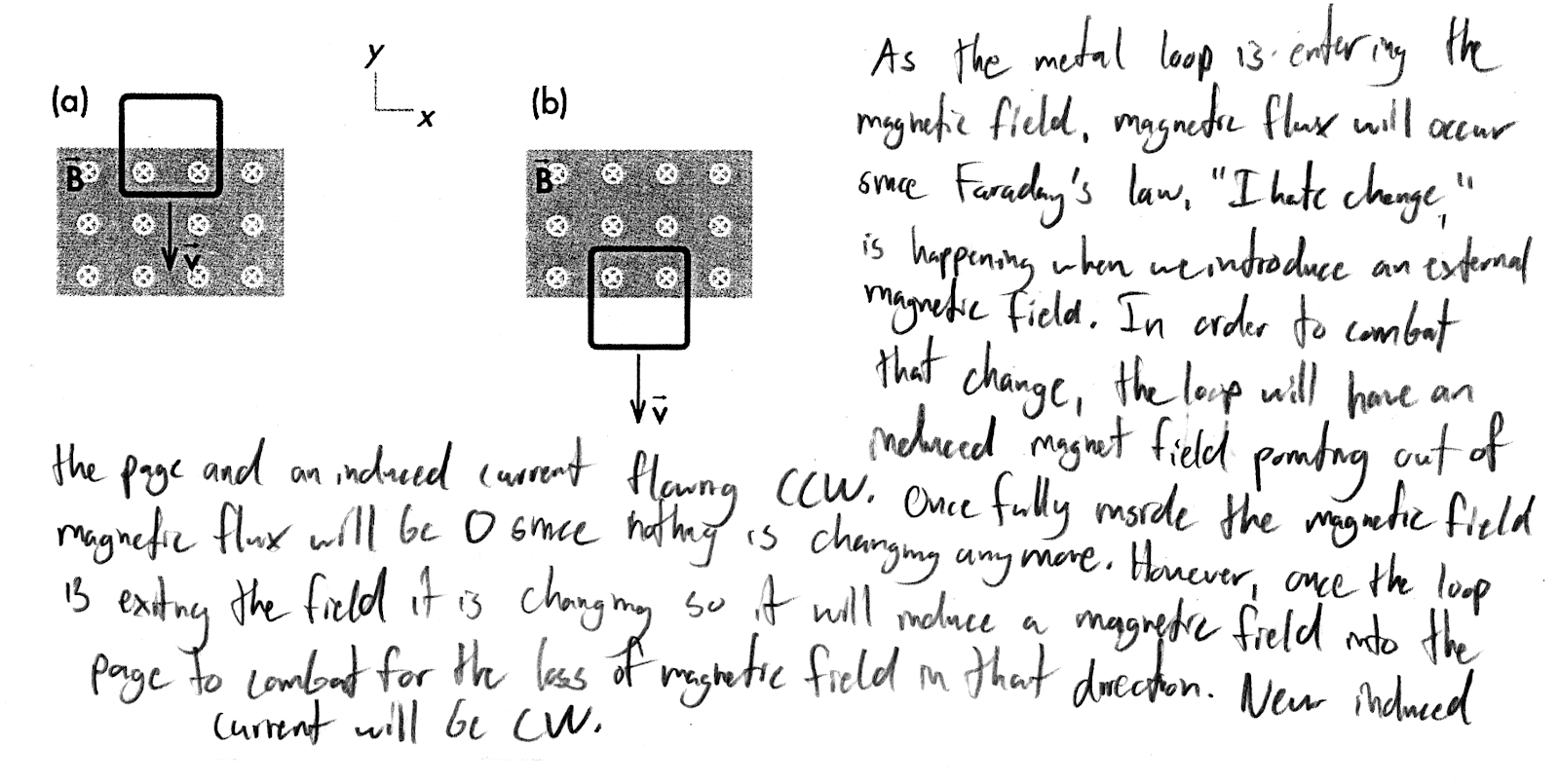## 20180505

### Physics midterm question: loop entering, leaving magnetic field

Physics 205B Midterm 2, spring semester 2018
Cuesta College, San Luis Obispo, CA

Within a certain region (shown in gray) there is an external uniform magnetic field, and the magnetic field is zero everywhere outside of this region. A square metal loop of resistance R moves with constant velocity as it enters, then exits this magnetic field region. Discuss why the induced current flows in one direction around the loop as it enters the magnetic field region, then flows in the other direction around the loop as it exits the magnetic field region. Explain your reasoning using the properties of magnetic fields, forces, motional emf, Faraday's law and Lenz's law.• p:
Correct. Explains how the induced current flows in one direction around the loop and in the other direction around the loop as it (a) enters and (b) exits the external magnetic field by using:
1. RHR1, where the velocity direction of the loop (downwards) and external magnetic field direction (pointing into the page) result in a force on the fictitious positive charges to the right in both cases (a)-(b), but only in the horizontal portion of the loop that is inside the magnetic field, thus resulting in an induced current that is counterclockwise in (a) and clockwise in (b); or
2. Faraday's law and Lenz's law, where moving the loop downwards would increase the magnetic flux going into the page through the loop in (a), and decreasing the magnetic flux going into the page through the loop in (b); and from Lenz's law, these changes in the magnetic flux through the loop will be "fought" by an induced current that must be flowing counterclockwise in (a), and clockwise in (b).
• r:
As (p), but argument indirectly, weakly, or only by definition supports the statement to be proven, or has minor inconsistencies or loopholes. Directions of induced current are clockwise in (a), and counterclockwise in (b), but otherwise still systematically applies RHR1 or Faraday's law and Lenz's law.
• t:
Nearly correct, but argument has conceptual errors, or is incomplete.
• v:
Limited relevant discussion of supporting evidence of at least some merit, but in an inconsistent or unclear manner. Some attempt at applying properties of magnetic fields, forces, motional emf, Faraday's law and Lenz's law.
• x:
Implementation of ideas, but credit given for effort rather than merit. No clear attempt at systematically applying properties of magnetic fields, forces, motional emf, Faraday's law and Lenz's law.
• y:
Irrelevant discussion/effectively blank.
• z:
Blank.
Sections 30882, 30883
Exam code: midterm02iFtW
p: 12 students
r: 12 students
t: 1 student
v: 5 students
x: 4 students
y: 0 students
z: 0 students

A sample "p" response (from student 1712):Another sample "p" response (from student 1929):